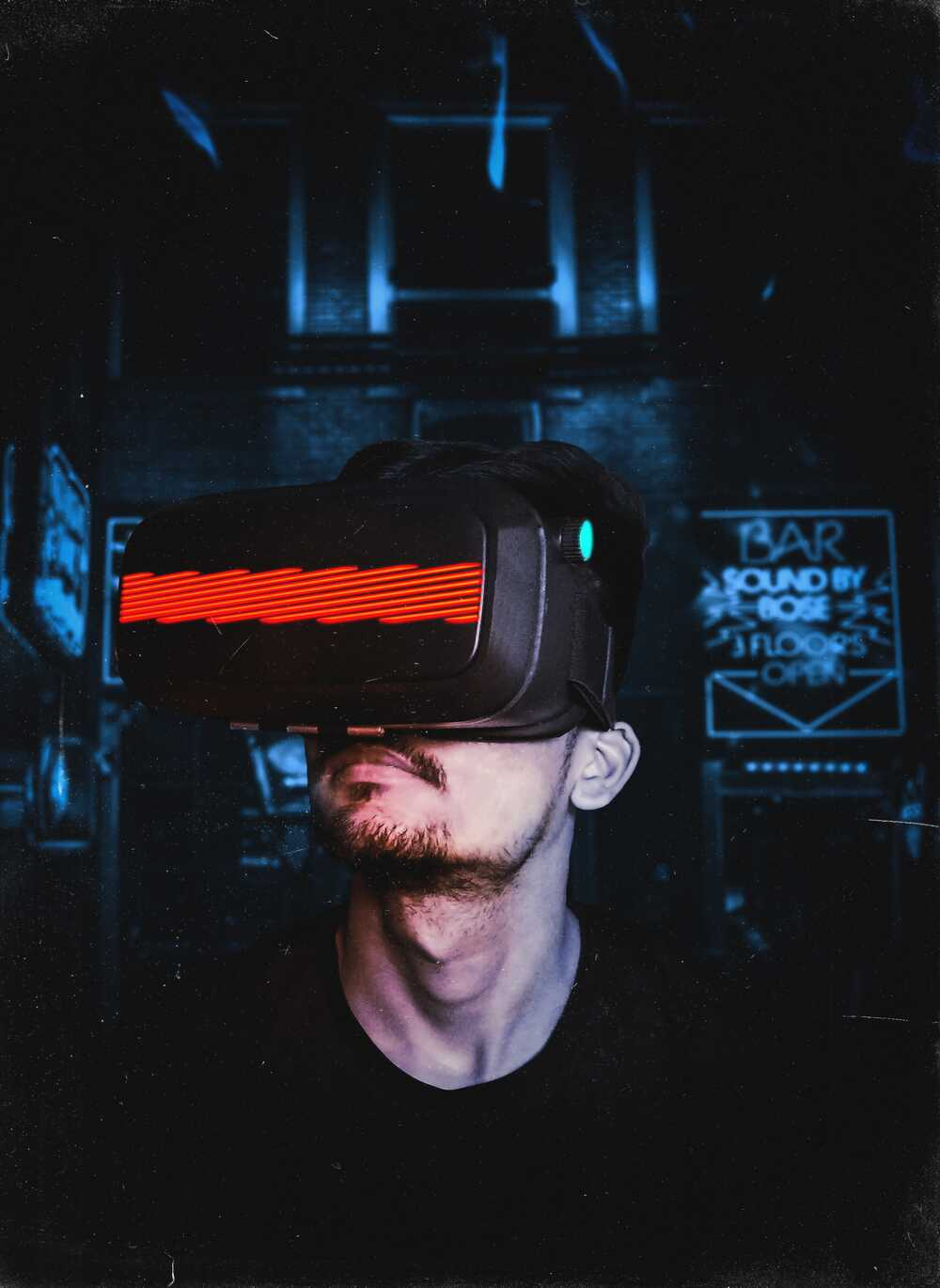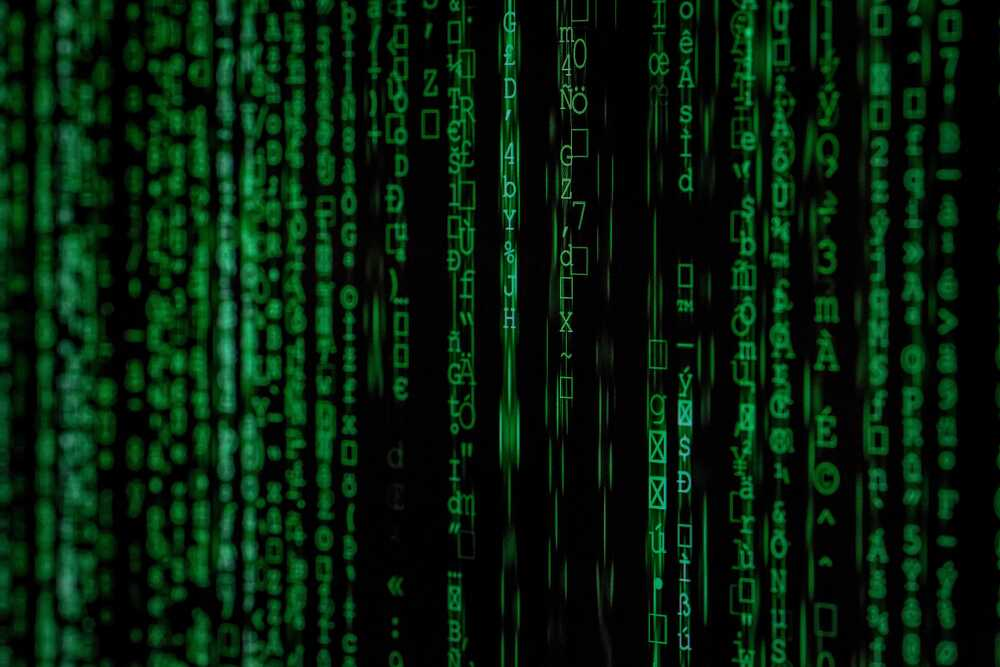# the future of the IT world?

The quantum computer is a great mystery at the moment since for many people it is just a myth. According to studies, this futuristic computer could become a reality. For now, this is only theoretical, but in a few years these theories could be put into practice by scientists. It is true that we are talking about this invention for years, but the more the years pass, the more we say to ourselves that this is possible. Especially now, if you look closely, the technology is improving and will improve even more in 4 years from now…

Let’s try to see beyond the tip of his nose with this article and understand the quantum world.## What does quantum mean?

There are several names that carry the quantum name like:

• quantum computer which can also be called “supercomputers”
• quantum computing is a step in the field of computer science that deals with quantum computers
• quantum mechanics is quite a complex mechanism and difficult to understand so in summary it is the quantum processor
• quantum logic is a logic with 3 factors (true, false and Heisenberg’s principle of indeterminacy)
• Quantum number is quantized values ​​that interpose in quantum states

Now what does the real meaning of quantum mean then quantum is a mechanism for behavioral processing of objects at the microscope level. This theory is a theory that has the function of describing discontinuous radiation and to know how to do this, it uses a concept called “quanta”. This phenomenon is called quantification. As an example of this phenomenon, the photoelectric effect can be used. In conclusion, we can say that it allows to calculate the probability of presence.

## What is the quantum internet?

The quantum internet which can simply be called great computer capable of calculationsvery sophisticated quantum mechanics and to convey messages in a complex world that is quantum mechanics. All this to say that this quantum internet has more complexity than just web applications. The quantum computer transforms its data into quantum states called qubits. Basically, the quantum device will send qubits into millions of quantum networks. A quantum state is the sending of qubit information to quantum channels.

To know how to use a quantum computer, you have to forget everything you know about the ordinary computer, because it doesn’t work the same way at all. Qubits are data that are superimposed and a phenomenon called superposition is found at the core of quantum mechanics. The problem with a quantum computer is that you can’t send messages in message apps like:

There is a protocol efficiency which is when the qubits propagate in the channel of the optical fiber and this means that the quantum signals have difficulty traveling long distances.

## How does a quantum computer work?

First, the quantum computer or supercomputer is used for computer calculations where the power of quantum calculations is impressive. The basis of its operation is the qubit which carries a superposition of 0 or 1. Indeed, it uses a superposition of several values. Basically, a quantum computer is 100,000 times more powerful than a classical computer, because the quantum PC will calculate 16 times faster than an ordinary PC.

Now there are quantum algorithms which are of course exponential. This makes it possible to search for things like phone numbers anywhere and anytime. In conclusion, qubits are very important in quantum computers.

## What is quantum physics for dummies?

Quantum physics is very complex to understand, but this paragraph will allow you to see it a little more clearly. Quantum physics is a quantum theory that allows working with laws or with quantum property.

The first thing to understand is the operation of an electron, a particle of light and molecules and of course, you have to be strong in equations. Physicists have discovered theories by experimenting with the quantum world. The quantum electron is, according to these theories, very versatile, because it behaves like a quantum cloud. If we try to measure a quantum electron, which is very difficult, we will immediately see without realizing that it uses the phenomenon of quantification and this electron will never stop.

According to the subatomic scale, the particles of light are very important there, because they are able to make electricity work and also gravity. The physicist at the origin of quantum physics is Albert Einstein who worked on a particular phenomenon: the phenomenon of entanglement. This phenomenon is used when two particles have the same theory of relativity and of course this relativity is included in the speed of light. A big mystery where scientists are currently working is the black ceramic mystery of the quantum world.

I’ quantum computer will have extraordinary faculties in complex calculations. Indeed in the construction of this computer, they will set up ideal properties capable of calculating to the thousandth of molecules. In conclusion, we are on the eve of a great technological revolution.

## What is a quantum object?

In the quantum world, there is also quantum objects which are very small. Indeed, a quantum object can take several quantum trajectories and this is called the phenomenon of quantum entanglement which can possess several objects by teleporting them without any delay and any connection. We are in an abstract quantum world which has fundamental mathematical importance, but we cannot deny that these are completely abstract theories. The interpretation of the theory of electro dynamism has a high level of the abstract. Indeed in this theory, we notice that the particles are charged at a distance.

## Conclusion

We are coming to the end of this article and I hope this one has taught you something about the quantum computer. For information, a start-up has just sold the very first quantum computer at a value of 5000 dollars. It’s a real computer revolution what we are going through. I hope this article made you understand the quantum world and thank you for reading.

Share